# Modules Generated by Sets of Submodules .... ....

Gold Member
MHB

## Homework Statement

I am reading Paul E. Bland's book "Rings and Their Modules" ...

Currently I am focused on Section 4.2 Noetherian and Artinian Modules ... ...

I need some help in order to make a meaningful start on Problem 1, Problem Set 4.1 ...

Problem 1, Problem Set 4.1 reads as follows: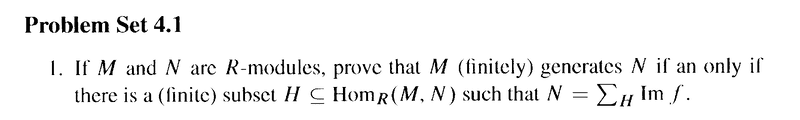Discussion of relevant definitions are given in my attempt at a solution ...

## The Attempt at a Solution

[/B]
Can someone please help me to make a meaningful start on Problem 1 above ... as well as clarifying the definition of one module generating another ... see my notes following ... ...

I am having a bit of trouble pinning down a definition from Bland that gives me the meaning of "a module ##M## generating a module ##N##" ...

Now ... on page 104 of Bland (see first page of Bland Section 4.1 below) we read the following:

" ... ... If ##\mathscr{S}## is a set of submodules of ##M## such that ##M = \sum_{ \mathscr{S} } N##, then ##\mathscr{S}## is said to span ##M## ... ... "

Now if spanning ##M## is the same as generating ##M## ... then in Problem 1 we could take ## \mathscr{S} = \{ M \} ## as generating ##N## ... but is that the correct interpretation of "span" and would the definition be useful in Problem 1 ... ?

Alternatively ... on page 105 we read in Definition 4.1.2 (see Bland's text displayed below ... )

" ... ... An R-module ##M## is said to be generated by a set ##\{ M_\alpha \}_\Delta ## of R-modules if there is an epimorphism ##\bigoplus_\Delta M_\alpha \rightarrow M##. ... ... "

Maybe this is the definition to use in Problem 1 above ... with ##\{ M_\alpha \}_\Delta = \{ N \}## ... ... is that the correct start on the problem ...?

Can someone please clarify the above ... and then, further, help me to make a meaningful start on the problem ...

Help will be appreciated,

Peter

==========================================================================================

In order to give readers the definitions, notation and context of Bland's treatment of generating and cogenerating classes, I am providing access to Bland's Section 4.1 ... as follows ...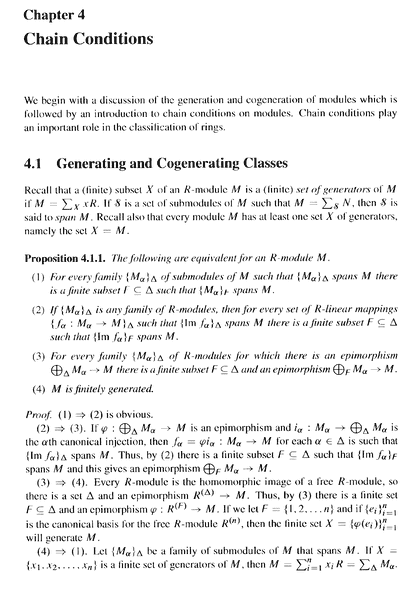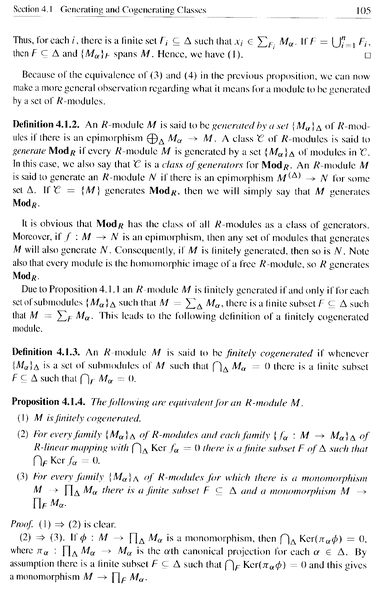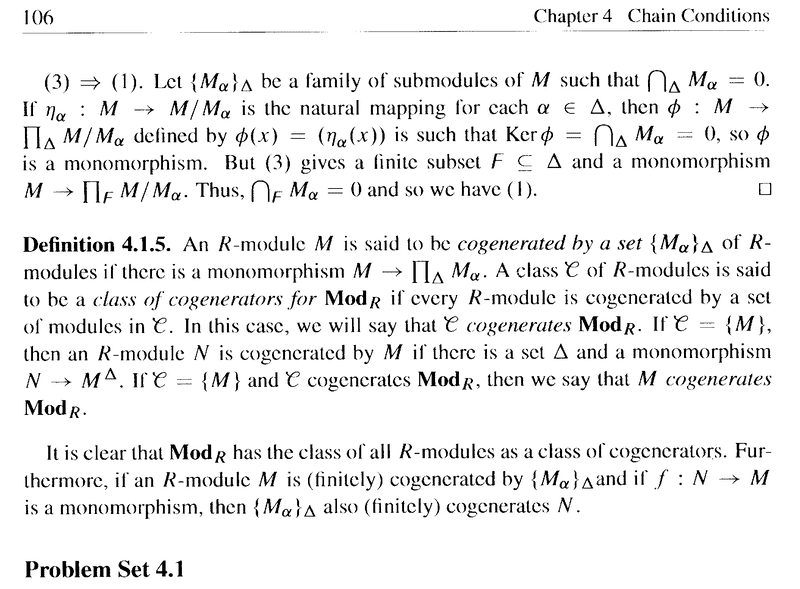Hope that helps ...

Peter

#### Attachments

Last edited:

Homework Helper
Gold Member
The two definitions are only algebraically different when the set of spanning/generating modules contains more than one module. A 'generating' set is a spanning set for which each pair of elements only has 0 in the intersection.

However the two definitions also have a set-theoretic difference, which is that in the 'spanning' definition the spanning modules must be submodules of the module they span, whereas in the 'generating' definition they do not have to be, and an epimorphism is invoked.

Since the claim that he asks us to proof does not say that ##M\subseteq N##, so we have to use the 'generates' definition (p105).

Now, I think the author's claim is wrong. Let ##N=\mathbb R^2## and ##M=\mathbb R##, and set ##H## to be the set of two homomorphisms ##f:x\mapsto(x,0)## and ##g:x\mapsto(0,x)##. Then ##N=\textrm{Im}\ f + \textrm{Im}\ g##. But there is no epimorphism from ##M## to ##N##. That would be a linear surjection from ##\mathbb R## to ##\mathbb R^2##, which I am pretty sure does not exist.

If that's correct then I think his definition of 'generates' needs tweaking. In particular, he needs to allow a module in the generating set to be used more than once in the direct sum. To do that would necessitate some additional construction.

•Math Amateur
Mentor
2022 Award
That would be a linear surjection from ##\mathbb R## to ##\mathbb R^2##, which I am pretty sure does not exist.
This depends on what you require ##\mathbb{R}^2## to be: the codomain or the image? ##\varphi = 0\, : \,\mathbb{R}\longrightarrow \mathbb{R}^2## is such an epimorphism, ##\mathbb{R}^2## isn't simply the image.

•Math Amateur
Homework Helper
Gold Member
This depends on what you require ##\mathbb{R}^2## to be: the codomain or the image? ##\varphi = 0\, : \,\mathbb{R}\longrightarrow \mathbb{R}^2## is such an epimorphism, ##\mathbb{R}^2## isn't simply the image.
I can't see a distinction to be made here. When we say that a map is a surjection then by definition we are saying that its codomain is the same as its image. So when we talk of a surjection from ##\mathbb R## to ##\mathbb R^2## we are saying that its image is ##\mathbb R^2##, which is all of its codomain.

Based on the terminology I was taught, that ##\varphi## example is not an epimorphism because its codomain ##\mathbb R^2## is different from its image ##\{0\}##. I understand that there are variations in how the terminology is used in this area, but I am not familiar with a variation in which that ##\varphi## would be considered an epimorphism.

•member 587159
Mentor
2022 Award
Yes, it's probably a tick of mine rather than the common notation. I use to think that the surjectivity of ##\varphi## in ##A \stackrel{\varphi}{\twoheadrightarrow } B \stackrel{\iota}{\hookrightarrow} C## is what counts and not ##\iota \varphi## if ##\iota## is a natural embedding. It allows to speak of epimorphisms into as being different from those onto.

Gold Member
MHB
Thanks for the help Andrew and fresh_42 ...

Where are we now ... has Bland actually asked us to prove an invalid statement...

Can you elucidate...

Peter

Homework Helper
Gold Member
The 'only if' direction is correct as written, and fairly straightforward.

For the 'if' direction, the definition of 'generated' needs to be changed. The following definition would work:

A module ##N## is said to be generated by a set ##\Delta## of ##R##-modules if there exists a nonnegative integer ##n##, a surjection ##\phi:\{k\in\mathbb Z\ :\ 1\le k\le n\}\to\Delta## and an epimorphism ##\psi:\bigoplus_{k=1}^n \phi(k)\to N##.​

The difference between this and the definition in the text is that it allows the use of multiple copies of some of the modules in ##\Delta##.

I should add that this is not my preferred definition of generated. I chose it because it is the closest one to the one in the text that allows the 'if' claim to be true.

I note that under this definition we cannot say that a module is generated by an infinite set of modules, which is something we may well want to say.

•Math Amateur
Gold Member
MHB
Thanks Andrew ...

Peter

Gold Member
MHB
The 'only if' direction is correct as written, and fairly straightforward.

For the 'if' direction, the definition of 'generated' needs to be changed. The following definition would work:

A module ##N## is said to be generated by a set ##\Delta## of ##R##-modules if there exists a nonnegative integer ##n##, a surjection ##\phi:\{k\in\mathbb Z\ :\ 1\le k\le n\}\to\Delta## and an epimorphism ##\psi:\bigoplus_{k=1}^n \phi(k)\to N##.​

The difference between this and the definition in the text is that it allows the use of multiple copies of some of the modules in ##\Delta##.

I should add that this is not my preferred definition of generated. I chose it because it is the closest one to the one in the text that allows the 'if' claim to be true.

I note that under this definition we cannot say that a module is generated by an infinite set of modules, which is something we may well want to say.

Hi Andrew ... still reflecting on what you have said ...

But ... still far from seeing a proof ...

Can you please show the main elements of a proof using your definition of generated ...

Peter

Homework Helper
Gold Member
What I had in mind for the 'if' case is the following:
Let ##\Delta=\{M\}## and let the designated finite subset ##H## of homorphisms from ##M## to ##N## be ##\{\theta_1,..., \theta_n\}##.
Then we use ##\mathscr M\triangleq\bigoplus_{k=1}^n M##, the external direct sum of ##h## distinct copies of ##M##, as the domain of our epimorphism ##\psi##.

Let ##L## be the subset (NB not submodule) of ##\mathscr M## made up of elements each of which has only one nonzero component. Define a map ##\zeta:L\to N## such that ##\zeta(m)=\theta_k(m_k)## where it is the ##k##th component of ##m## that is nonzero and that component is ##m_k##.

Then define ##\psi:\mathscr M\to N## to be the linear extension of the map ##\zeta## to a module homomorphism with domain ##\mathscr M##. The image of ##\psi## will be the sum of the images of all the ##\theta_k##, which is all of ##N##. The existence and uniqueness of such a linear extension is a fundamental theorem of modules, which I am presuming has already been proven earlier in the text.

In terms of the definition given earlier, the function ##\phi:\{1,...,n\}\to\Delta## is the constant function such that ##\forall k:\ \phi(k)=M##.

steenis
In cases like this, I always look in another book I have available. In this case “Anderson - Rings and Categories of Modules (2nd edition,1992)”:

This is the definition of Anderson (p.105):

(1) Let ##\mathscr{U} ## be a class of modules. A module ##M## is (finitely) generated by ##\mathscr{U} ## (or ##\mathscr{U} ## (finitely) generates ##M##) in case there is a (finite) indexed set ##(U_\alpha)_{\alpha \in A}## in ##\mathscr{U} ## and an epimorphism ##\bigoplus_A U_\alpha \to M##.

(2) If ##\mathscr{U} = \{U\} ## is a singleton, then we simply say that ##U## (finitely) generates M; ##\underline{ \text{of course} }## (!) this means that there is an epimorphism ##U^{(A)} \to M## for some (finite) set ##A##.

This is the definition of Bland (Definition 4.1.2.):

(1) An ##R##-module ##M## is said to be generated by a set ##(M_\alpha)_\Delta## of R-modules [[[ ##(M_\alpha)_\Delta## generates ##M## ]]] if there is an epimorphism ##\bigoplus_\Delta M_\alpha \to M##.

(2) An ##R##-module ##M## is said to generate an ##R##-module ##N## if there is an epimorphism ##M^{(\Delta)} \to N## for some set ##\Delta##.

Each of the definitions is clear, but I myself think it is not clear that (2) follows from (1), see “##\underline{ \text{of course} }##”. Maybe it should have been better explained by Bland.

Andrewkirk should have used definition (2) in stead of (1). In that case there is an epimorphism ##M^2 \to N##; but that is hindsight, it is certainly not clear at first sight. It is certainly understandable that definition (1) is interpreted in this way.

(Recall: If ##(M_\alpha)_\Delta## is a family of R-modules such that ##M_\alpha = M## for each ##\alpha \in \Delta## then ##\Pi_\Delta M_\alpha## and ##\bigoplus_\Delta M_\alpha## will be denoted by ##M^\Delta## and ##M^{(\Delta)}##, respectively; ##M^{(n)}## represents the direct product ##M \times M \times \cdots \times M## of ##n## factors of ##M##).

Maybe you also want to compare this with “Faith - Algebra I; Rings, Modules and Categories (1973)” on p.144 and “Rotman - Advanced Modern Algebra, part 2 (3rd,2017)” on p.388, but these definitions are in a categorical context.

Gold Member
MHB
What I had in mind for the 'if' case is the following:
Let ##\Delta=\{M\}## and let the designated finite subset ##H## of homorphisms from ##M## to ##N## be ##\{\theta_1,..., \theta_n\}##.
Then we use ##\mathscr M\triangleq\bigoplus_{k=1}^n M##, the external direct sum of ##h## distinct copies of ##M##, as the domain of our epimorphism ##\psi##.

Let ##L## be the subset (NB not submodule) of ##\mathscr M## made up of elements each of which has only one nonzero component. Define a map ##\zeta:L\to N## such that ##\zeta(m)=\theta_k(m_k)## where it is the ##k##th component of ##m## that is nonzero and that component is ##m_k##.

Then define ##\psi:\mathscr M\to N## to be the linear extension of the map ##\zeta## to a module homomorphism with domain ##\mathscr M##. The image of ##\psi## will be the sum of the images of all the ##\theta_k##, which is all of ##N##. The existence and uniqueness of such a linear extension is a fundamental theorem of modules, which I am presuming has already been proven earlier in the text.

In terms of the definition given earlier, the function ##\phi:\{1,...,n\}\to\Delta## is the constant function such that ##\forall k:\ \phi(k)=M##.

Hi Andrew,

You write:

" ... ... Let ##\Delta=\{M\}## and let the designated finite subset ##H## of homorphisms from ##M## to ##N## be ##\{\theta_1,..., \theta_n\}##.
Then we use ##\mathscr M\triangleq\bigoplus_{k=1}^n M##, the external direct sum of ##h## distinct copies of ##M##, as the domain of our epimorphism ##\psi##. ... ... "

Could you help by detailing the exact and explicit form of the ##\theta_i## and also ##\psi## ...

Peter

Homework Helper
Gold Member
Could you help by detailing the exact and explicit form of the ##\theta_i## and also ##\psi## ...
Peter
We don't know anything about the form of the ##\theta_k##other than that they are homomorphisms from ##M## to ##N##, but we know they exist because for this direction of the proof we start with the premise that there is a finite subset ##H\subseteq \mathrm{Hom}(M,N)## such that ##N = \sum_H \mathrm{Im}\ f ##. That's the author's statement - see the statement of Problem 4.1. All I've done is label the elements of ##H## as ##\theta_1,...,\theta_n## where ##n=|H|##, rather than use the undefined ##f## that the author uses. So in my notation we'd write that premise as ##N = \sum_{k=1}^n \mathrm{Im}\ \theta_k ##.

##\psi## is just the linear extension of ##\zeta##, expanding the domain from ##L## to ##\mathscr M##. So for ##m=(m_1,m_2,...,m_n)\in\mathscr M## we have
$$\psi(m) = \sum_{k=1}^n \theta_k(m_k)$$

•Math Amateur
Gold Member
MHB
The 'only if' direction is correct as written, and fairly straightforward.

For the 'if' direction, the definition of 'generated' needs to be changed. The following definition would work:

A module ##N## is said to be generated by a set ##\Delta## of ##R##-modules if there exists a nonnegative integer ##n##, a surjection ##\phi:\{k\in\mathbb Z\ :\ 1\le k\le n\}\to\Delta## and an epimorphism ##\psi:\bigoplus_{k=1}^n \phi(k)\to N##.​

The difference between this and the definition in the text is that it allows the use of multiple copies of some of the modules in ##\Delta##.

I should add that this is not my preferred definition of generated. I chose it because it is the closest one to the one in the text that allows the 'if' claim to be true.

I note that under this definition we cannot say that a module is generated by an infinite set of modules, which is something we may well want to say.

Hi Andrew ... thanks again for all your explanations and help ...

But just a further clarification on your idea of changing the definition of generated ...

You write:

" ... ... For the 'if' direction, the definition of 'generated' needs to be changed. The following definition would work:

A module ##N## is said to be generated by a set ##\Delta## of ##R##-modules if there exists a nonnegative integer ##n##, a surjection ##\phi:\{k\in\mathbb Z\ :\ 1\le k\le n\}\to\Delta## and an epimorphism ##\psi:\bigoplus_{k=1}^n \phi(k)\to N##.​

The difference between this and the definition in the text is that it allows the use of multiple copies of some of the modules in ##\Delta##. ... "

But ... where and in what way does Bland forbid the use of multiple copies of some of the modules ...?

He certainly allows multiple copies of the same module when he writes ( ... near the end of Definition 4.1.2 ... )

" ... ... An R-module ##M## is said to generate an R-module ##N## if there is an epimorphism ##M^{ ( \Delta ) } \rightarrow N## for some set ##\Delta## ... "

The notation ##M^{ \Delta }## is explained by Bland in his section on free modules ... see under "Notation" in the following: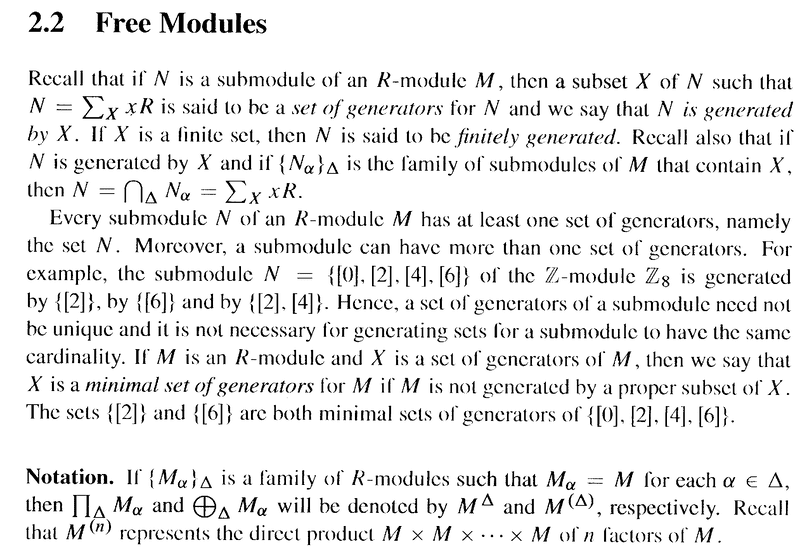Just a further note on your amended definition ...

You appear to have taken Bland's definition given at the start of Definition 4.1.2 ... namely ...

" ... ... An R-module ##M## is said to be generated by a set ##\{ M_\alpha \}_\Delta## of R-modules if there is an epimorphism ##\bigoplus_\Delta M_\alpha \rightarrow M##. ... ... "

and added the finite generation condition of Proposition 4.1.1 part (3) ... ... (see below) ...

Can you comment? ... am I misunderstanding you (my apologies in advance if I am ... ) ... also some of these points may have been covered by steenis ... I am still reflecting on what he has written ...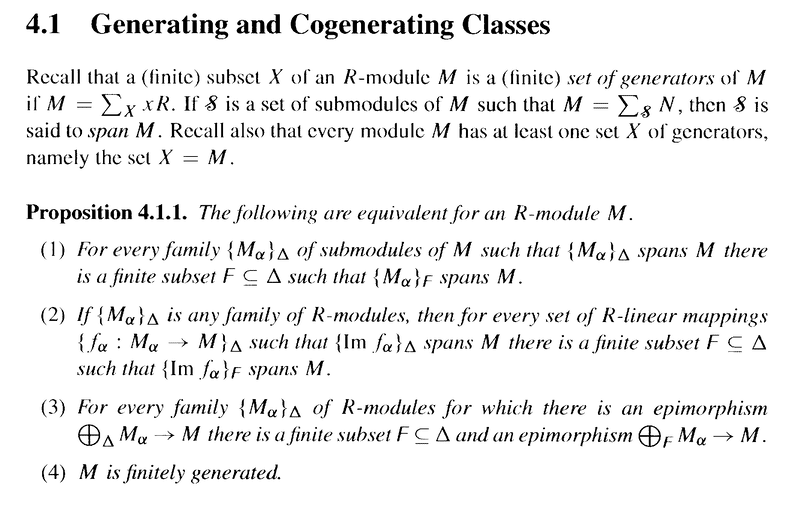It may be useful to you to have a copy of Definition 4.1.2 available ... so I am providing the same ... as follows: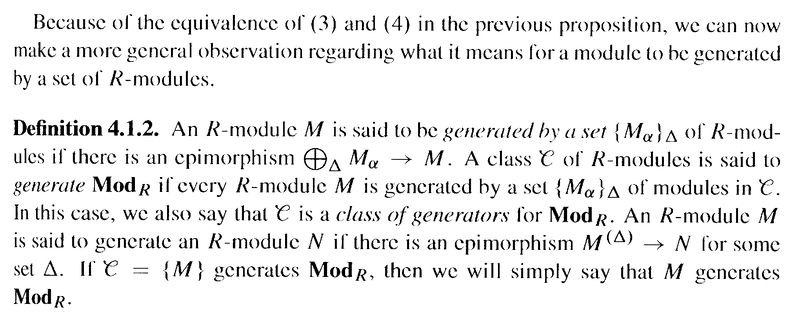Peter

#### Attachments

Last edited:
Homework Helper
Gold Member
That definition under heading 'Notation' in section 2.2 is new information, and it gives a different meaning to the excerpts quoted in the OP. With that new interpretation, his definition now means the same as mine.

It is nonstandard notation that I have not seen it used by anybody else, and it seems perilously misleading to me because it looks as though ##\bigoplus_{\Delta} M## is meant to read ##\bigoplus_{M\in\Delta} M## which definitely doesn't allow recycling summands, just as ##\sum_{k\in\{1,2,3\}}k## is ##1+2+3=6## and not ##1 + 1+ 2+ 2+ 2+ 3 = 11##.

But an author is entitled to make up whatever notation they like in their own book.

•Math Amateur
Gold Member
MHB
That definition under heading 'Notation' in section 2.2 is new information, and it gives a different meaning to the excerpts quoted in the OP. With that new interpretation, his definition now means the same as mine.

It is nonstandard notation that I have not seen it used by anybody else, and it seems perilously misleading to me because it looks as though ##\bigoplus_{\Delta} M## is meant to read ##\bigoplus_{M\in\Delta} M## which definitely doesn't allow recycling summands, just as ##\sum_{k\in\{1,2,3\}}k## is ##1+2+3=6## and not ##1 + 1+ 2+ 2+ 2+ 3 = 11##.

But an author is entitled to make up whatever notation they like in their own book.

My apologies Andrew ... I should have provided an explanation to the notation before now ...

Thanks again for all your help ... I'd definitely be lost without your help ...

Peter

steenis
That definition under heading 'Notation' in section 2.2 is new information, ...
.

This definition was already posted in post #11 ...

steenis
This question still has no answer, so here it is:

To prove: ##M## and ##N## are R-modules.
##M## (finitely) generates ##N## ##\Leftrightarrow## there is a (finite) subset ##H \subset \text{Hom }(M, N)## such that ##N = \Sigma_H \text{im } f##.

Recapitulation
Definition 4.1.2. of Bland
(1) An ##R##-module ##M## is said to be generated by a set ##(M_\alpha)_\Delta## of R-modules [[[ ##(M_\alpha)_\Delta## generates ##M## ]]] if there is an epimorphism ##\bigoplus_\Delta M_\alpha \to M##.
(2) An ##R##-module ##M## is said to generate an ##R##-module ##N## if there is an epimorphism ##M^{(\Delta)} \to N## for some set ##\Delta##.

Proposition 2.1.5 of Bland
If ##\{M_\alpha | \alpha \in \Delta\}## is a family of R-modules, then the direct sum ##(\bigoplus_\Delta M_\alpha, i_\alpha)##,
has the property that for
every ##R##-module ##N## and
every family ##\{\phi_\alpha:M_\alpha \to N | \alpha \in \Delta\}##
there is a unique R-map ##\phi:\bigoplus_\Delta M_\alpha \to N##
such that ##\phi \circ i_\alpha = \phi_\alpha##, for all ##\alpha \in \Delta##, where the ##i_\alpha## are the canonical injections ##i_\alpha:M_\alpha \to \bigoplus_\Delta M_\alpha##, for ##\alpha \in \Delta##,

(Recall: If ##(M_\alpha)_\Delta## is a family of R-modules such that ##M_\alpha = M## for each ##\alpha \in \Delta## then ##\Pi_\Delta M_\alpha## and ##\bigoplus_\Delta M_\alpha## will be denoted by ##M^\Delta## and ##M^{(\Delta)}##, respectively.

Proof:
##\Rightarrow##) ##M## generates ##N## so there is an (index-) set ##\Delta## and an epimorphism ##f:M^{(\Delta)} = \bigoplus_\Delta M_\alpha \to N## (where ##M_\alpha = M##) by definition 4.1.2.(2).
For each ##\alpha \in \Delta## define ##f_\alpha = f \circ i_\alpha##, where ##i_\alpha: M_\alpha = M \to \bigoplus_\Delta M_\alpha ## is the canonical inclusion.
Now ##f_\alpha \in \text{Hom }(M, N)##. Define ##H = \{f_\alpha:M_\alpha = M \to N | \alpha \in \Delta \}##.
To prove: ##N = \Sigma_H \text{ I am } f##.

Let ##y \in N##, then there is a ##(x_\alpha)_\Delta \in \bigoplus_\Delta M_\alpha## such that ##y = f((x_\alpha)_\Delta) = \Sigma_\Delta f_\alpha(x_\alpha)## by prop.2.1.5. and because ##f## is an epimorphism.
We have ##f(x_\alpha) \in \text{ I am } f_\alpha##, for ##\alpha \in \Delta##.
Thus ##N \subset \Sigma_H \text{ I am } f##, therefore ##N = \Sigma_H \text{ I am } f##.

##\Leftarrow##) There is a subset ##H \subset \text{Hom }(M, N)## such that ##N = \Sigma_H \text{ I am } f##.
Let ##H = \{h_\alpha:M \to N | \alpha \in \Delta \}##.
Define ## f:M^{(\Delta)} = \bigoplus_\Delta M_\alpha \to N## (where ##M_\alpha = M##) by ##f = \Sigma_\Delta f_\alpha##, that is:
##f((x_\alpha)_\Delta) = \Sigma_\Delta f(x_\alpha)## for ##((x_\alpha)_\Delta \in \bigoplus_\Delta M_\alpha ##. To prove: f is an epimorphism.

Let ##y \in N## then ##y \in \Sigma_H \text{ I am } f = \Sigma_\Delta \text{ I am } f_\alpha##.
Then there are ##x_\alpha \in M_\alpha = M## such that ##y = \Sigma_\Delta f_\alpha(x_\alpha)##.
Then ##f((x_\alpha)_\Delta) = \Sigma_\Delta f_\alpha(x_\alpha) = y## by prop.2.1.5 and therefore f is surjective.

•Math Amateur